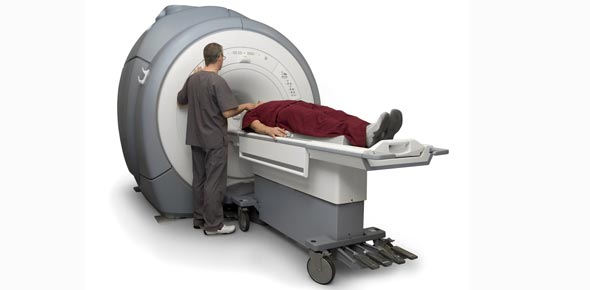# MRI 2

29 Questions | Attempts: 1641
ShareSettings• 1.
At a field strength of 1.0 tesla, the approximate T2 relaxation time for grey matter is what?
• A.

100MS

• B.

80MS

• C.

90MS

• 2.
In a T2 weighted image, CSF has a ________ T2 relaxation time and therefore appears _______.
• A.

Long, Bright

• B.

Long, Dark

• C.

Short, Dark

• D.

Short, Bright

• 3.
At a field strength of 1.0 tesla, the approximate T2 relaxation time for renal cortex tissue is what?
• A.

45MS

• B.

50MS

• C.

60MS

• D.

70MS

• 4.
At a field strength of 1.0 tesla, the approximate T2 relaxation time for spleen tissue is what?
• A.

60MS

• B.

80MS

• C.

90MS

• D.

100MS

• 5.
When the phase encoding gradient is activated, steep slopes produce what type of signal amplitude?
• A.

LOW

• B.

HIGH

• C.

MEDIUM

• D.

STRONG

• 6.
A 180 degree RF pulse is used to ______ the dephasing net vector in the transverse plane.
• A.

MAGNETIZE

• B.

REFOCUS

• C.

DECAY

• D.

DELAY

• 7.
At a field strength of 1.0 tesla, the approximate T1 relaxation time for CSF is what?
• A.

800MS

• B.

1000MS

• C.

2000MS

• D.

2500MS

• 8.
At a field strength of 1.0 tesla, the approximate T2 relaxation time of fat is what?
• A.

40

• B.

50

• C.

80

• D.

90

• 9.
During the acquisition of axial images of the body with the phase direction A/P, frequency encoding is performed by which physical gradient?
• A.

X

• B.

Y

• C.

Z

• 10.
At a field strength of 1.0 tesla, the approximate T1 relaxation time for muscle tissue is what?
• A.

270

• B.

480

• C.

600

• D.

680

• 11.
In a T1 weighted image, a short TR is used to do what?
• A.

MAX T2

• B.

MIN T2

• C.

MAX T1

• D.

MIN T2

• 12.
At a field strength of 1.0 tesla, the approximate T2 relaxation time for renal medulla tissue is what?
• A.

90

• B.

100

• C.

140

• D.

300

• 13.
During the acquisition of axial images of the body with the frequency direction L/R, phase encoding is performed by which physical gradient?
• A.

X

• B.

Y

• C.

Z

• 14.
In a T2 weighted image, tissues with long T2 relaxation times appear ______.
• A.

HYPERINTENSE

• B.

HYPOINTENSE

• C.

ISOINTENSE

• 15.
Which of the following logical gradients is known as the phase encoding gradient?
• A.

X

• B.

Y

• C.

Z

• 16.
The range of frequencies that is sampled during frequency encoding is known as what?
• A.

RECIEVE BANDWITH

• B.

TRANSMIT BANDWITH

• C.

• D.

RF SLOPE

• 17.
The Y gradient alters the magnetic field strength along which axis?
• A.

HORIZONTAL

• B.

VERTICAL

• C.

LONG

• D.

SHORT

• 18.
During slice selection, the Y gradient is used to select slices in which plane?
• A.

CORONAL

• B.

AXIAL

• C.

SAGITTAL

• 19.
At a field strength of 1.0 tesla, the approximate T1 relaxation time for spleen tissue is what?
• A.

360

• B.

380

• C.

400

• D.

480

• 20.
At a field strength of 1.0 Tesla, the approximate T1 relaxation time for blood is what?
• A.

540

• B.

680

• C.

800

• D.

1000

• 21.
The image parameter that primarily affects T1 weighting is known as what
• A.

TE

• B.

TR

• C.

IR

• 22.
At a field strength of 1.0 tesla, the approximate T1 relaxation time for renal medulla tissue is what?
• A.

520

• B.

600

• C.

680

• D.

800

• 23.
At a field strength of 1.0 tesla, the approximate T1 relaxation time for renal cortex tissue is what?
• A.

80

• B.

270

• C.

360

• D.

390

• 24.
The X gradient alters the magnetic field strength along which axis
• A.

HORIZONTIAL

• B.

VERTICAL

• C.

LONG

• D.

SHORT

• 25.
The time between the 90 degree RF pulse and the spin echo signal in a spin echo pulse sequence is known as what?
• A.

TR

• B.

TE

• C.

INVERSION TIME

• D.

RELAXATION TIME

## Related TopicsBack to top
×

Wait!
Here's an interesting quiz for you.